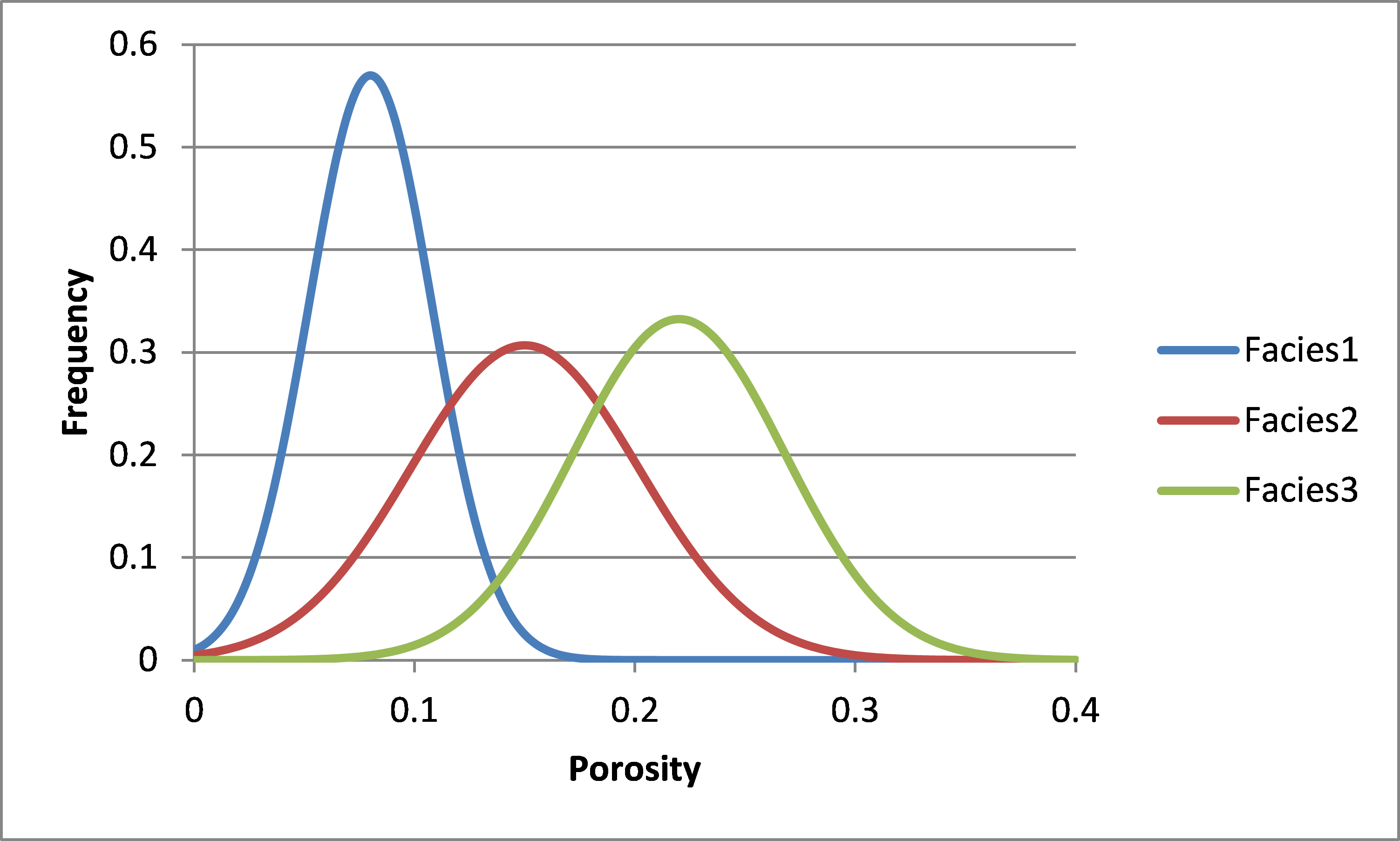# Consulting Corner: Enhancing Facies proportions model for a more realistic geological model

January 11, 2018

All the Facies modelling methods are using Facies proportions spatial models as soft constraints to enhance geological models realism. The use of such soft constraints strongly guides the Facies propagation in the interwell space. Therefore, it is of primary importance to build a Facies proportions distribution which is accurate and realistic.

This goal can be reached by integrating several sources of data of different types and different scales, all being correlated with the Facies proportions, such as well and seismic informations.

There are several methods to propagate expected Facies proportions from wells (where these proportions are known) into the 3D space, considering seismic data as constraints. Usually, seismic attibutes are used as drift or auxiliary variable in cokriging or cosimulations calculations. Another idea consists in combining seismic attributes transformed into Porosity, calibrated to well data, with the Porosity distribution per Facies determined from core plugs and Porosity logs.

For this last approach, the workflow is made of two steps, the first one corresponding to calculations at seismic vertical scale, the second one being a resolution enhancement at well data scale (between 1 meter and 1 foot).

At the seismic vertical scale, the average Porosity at a given location in the reservoir is obtained from a mixture of already defined Facies, which expected proportions are related to the Porosity distribution inside each Facies.It is not difficult to calculate the possible combinations of facies proportions and to calculate, for each combination, the expected Porosity, accounting for the Porosity distribution in each facies. Then, for a given average Porosity somewhere in the reservoir (derived from seismic data), with a given uncertainty range, it is easy to define the corresponding possible facies proportions combinations at that location. It is also easy to extract, for each facies, the corresponding most likely Porosity value and the associated Porosity variance. These most likely proportion values and the corresponding variances can be included as uncertain data in a kriging with measurement error, the reliable data being the Facies proportions at wells calculated by upscaling at seismic vertical scale. Then, Facies Proportions model honouring well data, strongly influenced by seismic data and accounting for petrophysical measurements can be generated.

To determine the vertical evolution of Facies proportions at a metric or sub-metric scale, it is possible to use these results at seismic scale as an input to the Proportions mapping algorithm implemented in Isatis, which ensures that, within a given vertical interval, the mean of estimated proportions (at high resolution) equals a user-defined proportion value (the estimated value at seismic scale).